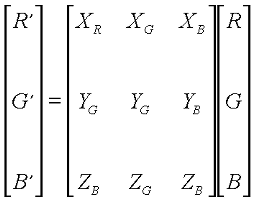# Color Conversion

### To convert from one color gamut to another is a simple procedure. Each phosphour color can be represented by a combination of the CIE XYZ primaries, yielding the following transformation from RGB to CIE XYZ:C = 1 - R
M = 1 - G
Y = 1 - B

### The fourth color, K, can be used to replace equal amounts of CMY:

K = min(C,M,Y) C' = C - K
M' = M - K
Y' = Y - K

 Web www.vrarchitect.net